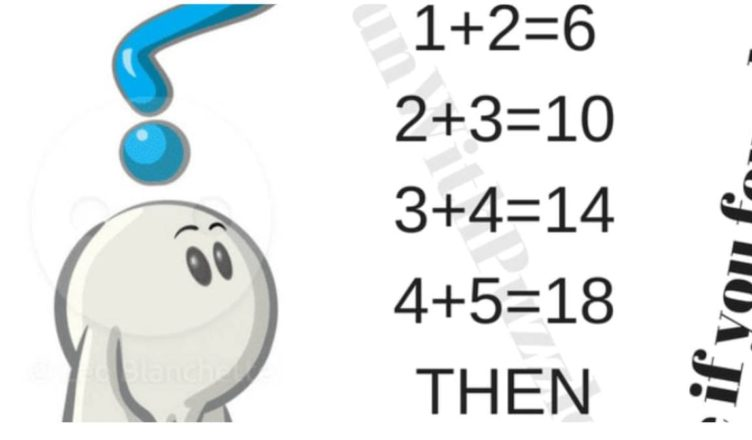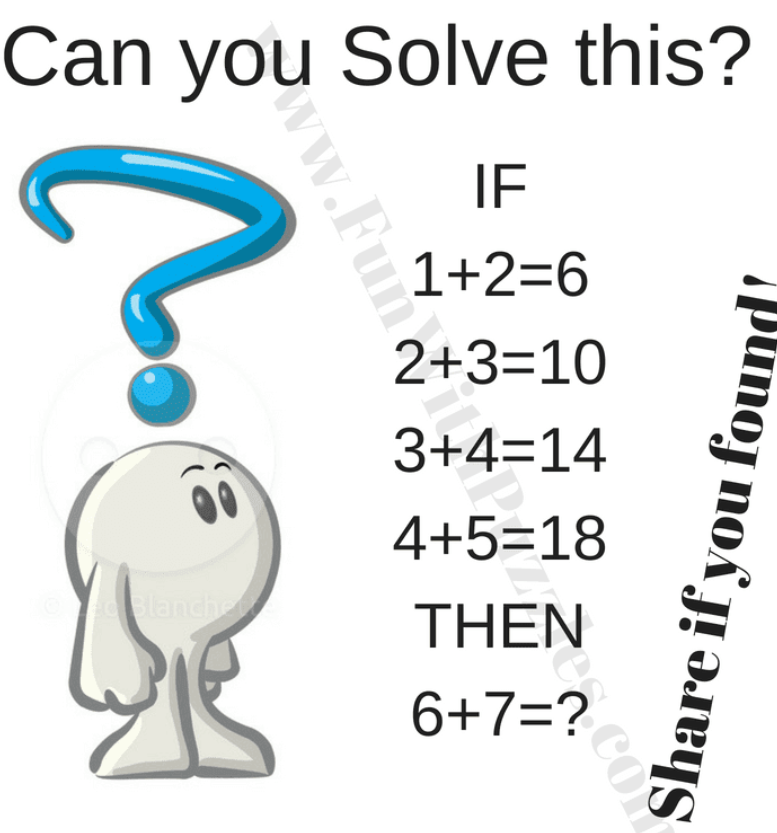# Brain Test Question.Here is an easy brain test question. There are many puzzles that are difficult to solve, but this one seems almost impossible. Is this puzzle really as difficult as everyone said? If you still cannot solve it,

you can also find the answer and the process on the Internet. So can you find the logical reasoning which makes these equations correct and then find the missing number which will the solution of the last equation in the given puzzle picture?In this Brain Test, there is some number equation. Each of these number equations follows the same logic which makes these equations correct.

There is no better way to test your IQ than to solve a puzzle, and see how well you do. There is a puzzle that is taking the Internet by storm and leaving those who cannot solve it perplexed. The answer is 26. If A+B=C is the given logical ebullition: 2*(A+B)=C.